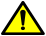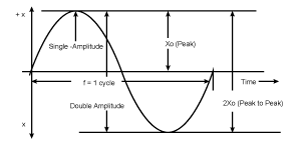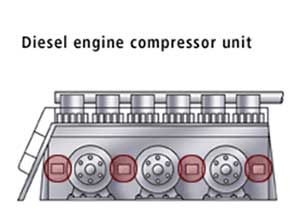WARNING: Cancer and Reproductive Harm - www.P65Warnings.ca.gov

# UNDERSTANDING THE PHYSICAL CHARACTERISTICS OF VIBRATION

## Introduction

Many people are aware that the amplitude (displacement) of a machine, resulting from a vibrating force, is a critical factor in causing mechanical damage to operating machinery. But many people are not aware that the FREQUENCY at which the vibratory force is occurring is of even greater importance in causing mechanical damage.

In general terms, vibration can be described as ". . . mechanical motion or oscillation about a reference point of equilibrium." To some this may only mean "movement", while to others it might suggest both movement and the relative number of movements per unit of time. To most people it may help to describe, on an operating machine, a condition indicating impending danger when the "vibration" appears to be exceeding a level previously considered normal.

## Definitions of Terms

Three simple terms are most frequently used in discussing vibration:

1. Displacement Also known as "amplitude" is defined as the magnitude of the motion under study, generally measured as peak-to-peak or double amplitude in "mils" (thousandths of an inch). These measurements are most important in the study of deformation or bending of structures.

2. Velocity The time rate of change of displacement, which is the first derivative of displacement with respect to time. Velocity measurements are most important where the noise from vibration is of greatest importance.

3. Acceleration The second derivative of displacement with respect to time. Its magnitude is proportional to displacement and the square of the frequency. These measurements are most practical and important where actual mechanical failure of parts is possible. The unit of measurement is "gravity" abbreviated by the letter "G". The formula is G = KAF**2, where
K - is a numerical constant
A - is the amplitude of motion
F - is the frequency at which the amplitude (displacement) is occurring.

Note that the total G forces (the destructive shock acting upon the bearings, shafts. etc.) varies directly with the amplitude (A) but with the SQUARE of the frequency (F). Therefore, doubling the amplitude (A) will double the destructive shock; while doubling the frequency (F) will increase the destructive shock by four times.

## WHY MONITOR ACCELERATORY VIBRATION?

Figure 1 shows the resultant wave form of displacement with respect to time of a pure harmonic motion producing a sinusoidal single frequency wave form. Robertshaw vibration instruments measure the total acceleratory forces (shock) and are therefore responsive to both amplitude and frequency of the resultant vibration. Vibration instruments that are responsive only to amplitude (displacement) are unable to detect the total destructive forces" (i.e. "G's") that will tear costly equipment apart.### It is important to remember these points:

1) The fact that a particular point on a machine is being displaced offers little information in regards to its destructive forces.

2) Acceleration measured at a particular point on a machine indicates the TOTAL vibratory shock and, therefore, offers a useful figure of merit of the total destructive forces present.

3) Operating machines' vibrations seldom follow pure harmonic motions at a single frequency, but rather they are combinations of a fundamental frequency (basic machine speed) and a number of higher harmonic frequencies. Since such vibrations result in a complex wave form (non-sinusoidal), acceleration sensing instruments offer the best measure of these destructive vibratory forces

## TYPICAL INSTALLATION ON A MACHINE

Typically the vibration switch should be placed as close to the bearing assemblies, crank shafts, driven gears and other rotation or reciprocating

Cooling Tower FanEngine Gear CompressorDiesel Engine CompressorCentrifugal PumpCompressor